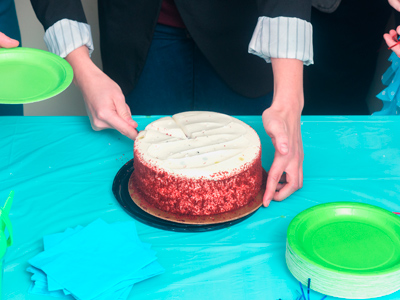Even numbers can be shared equally by two people, without having to cut them in half!

# Year 1 Numbers - Odd or Even

This quiz addresses the requirements of the National Curriculum KS1 Maths and Numeracy for children aged 5 and 6 in year 1. Specifically this quiz is aimed at the section dealing with recognising odd or even numbers.

Recognising odd or even numbers is an important early number skill. It is one of the first properties of number children will be taught to recognise. Knowing odd and even will help children in other areas of their maths development, including dividing numbers and completing sequences.

Question 1
Odd numbers end with the digits:
1, 2, 3, 4 or 5
1, 3, 5, 7 or 9
0, 1, 2, 4 or 6
2, 4, 6, 8 or 0
The last digit of an odd number is always one of these
Question 2
Even numbers always end with:
2, 3, 4, 5 or 6
1, 3, 5, 7 or 9
1, 2, 3, 4 or 5
2, 4, 6, 8 or 0
The last digit of an even number is always one of these
Question 3
Which of the following numbers is odd?
17
16
14
12
The last digit is a 7, which makes the number odd
Question 4
Which of the following sets of numbers are all odd?
2, 3, 4
10, 20, 30
11, 17, 21
12, 13, 14
All of these numbers end with an odd digit
Question 5
Which of these numbers is even?
17
18
15
13
The last digit is an 8, which makes this number even
Question 6
Which of the following sets of numbers are all even?
12, 13, 15
20, 22, 24
2, 4, 7
21, 23, 25
The last digit in each of these numbers is even, making the number even
Question 7
Which statement is true?
Even numbers can all be divided exactly by 2
Even numbers are always more than 20
Even numbers sometimes end with '3'
Even numbers always end with 2
Even numbers can be divided by two to give a whole number as the answer
Question 8
Which statement is true?
Odd numbers are all in the two times table
Odd numbers can end with a 2 or 4
Odd numbers are always bigger than even numbers
The last digit in an odd number is always 1, 3, 5, 7 or 9
Odd numbers always end with one of these digits, no matter how big they are
Question 9
Which set has an odd number in it?
2, 4, 6, 8, 10
2, 3, 4, 6, 8
6, 8, 10, 12, 14
10, 20, 30, 40, 50
3 is an odd number
Question 10
Which set has an even number in it?
1, 3, 5, 7, 9
31, 33, 35, 37, 39
23, 25, 27, 29, 31
13, 14, 15, 17, 19
14 is an even number
Author:  Angela Smith